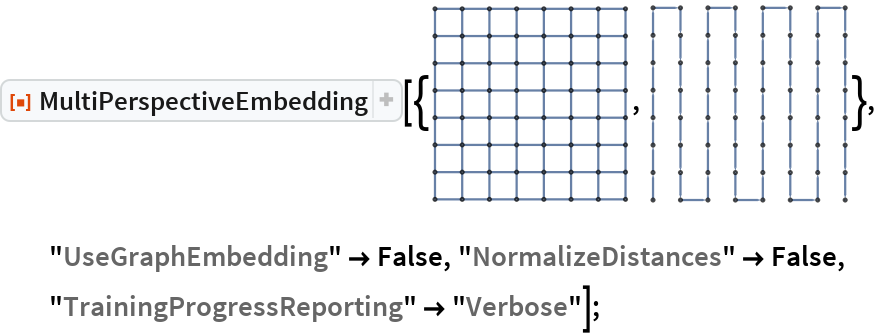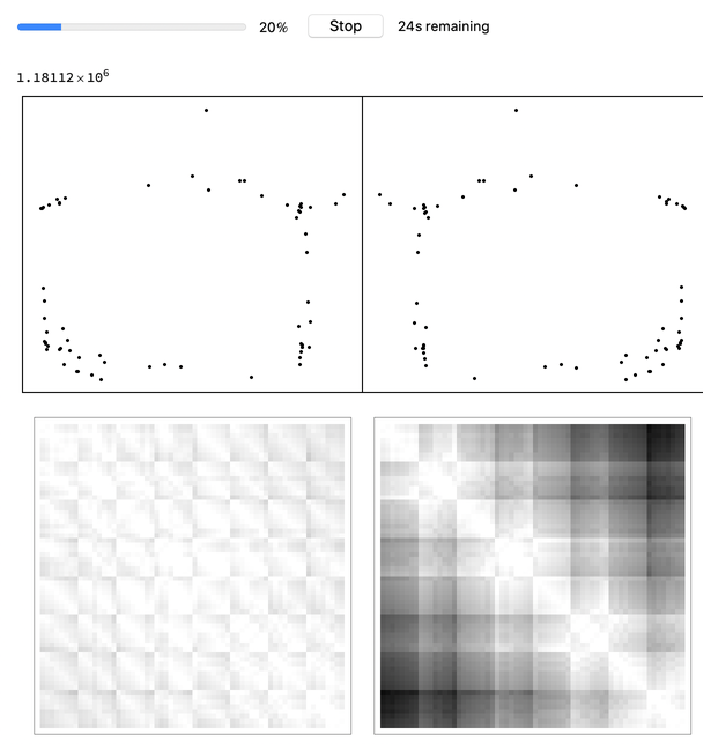#Function Repository Resource:

# MultiPerspectiveEmbedding

Compute a 3D embedding for multiple graphs so that all pairwise distances are preserved simultaneously along various 2D projections

Contributed by: Nikolay Murzin
 ResourceFunction["MultiPerspectiveEmbedding"][gs] gives multi-perspective 3D embedding of graphs gs. ResourceFunction["MultiPerspectiveEmbedding"][ds] gives multi-perspective 3D embedding for explicit distance matrices.

## Details and Options

The Graph inputs gs should have the same set of vertices.
Distance matrices ds should be square and of the same dimensions.
ResourceFunction["MultiPerspectiveEmbedding"] accepts the following options:
 "InitialEmbedding" array of initial embedding vectors "InitialProjection" array of initial normal vectors "FixProjection" whether to fix or learn projection vectors "UseGraphEmbedding" if input are graphs, whether to use its embeddings for computing distances "NormalizeDistances" whether to normalize distances "NetTrainOptions" {} custom options for NetTrain use "Verbose" for custom reporting
This is an elementary layer for training embeddings X, with one projection normal vector Q and a distance matrix D.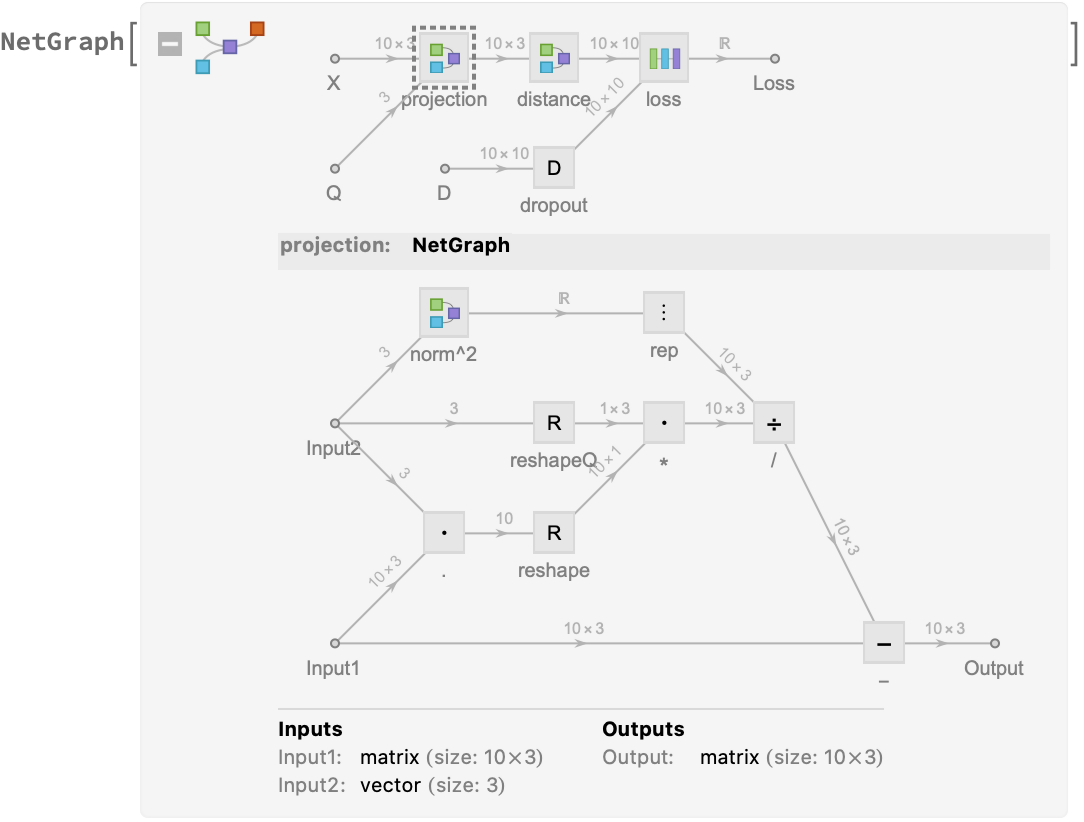## Examples

### Basic Examples

Compute a 3D embedding for a grid graph and a path graph:

 In:=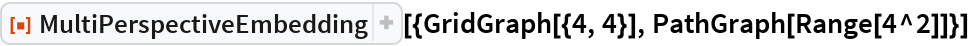Out=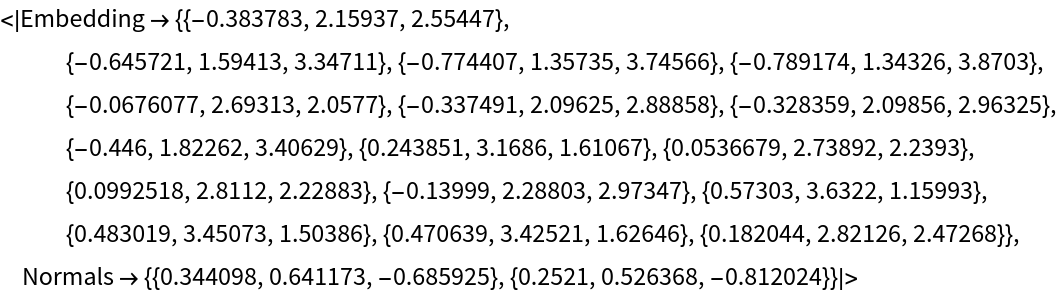Define larger grid and path graph and show them:

 In:=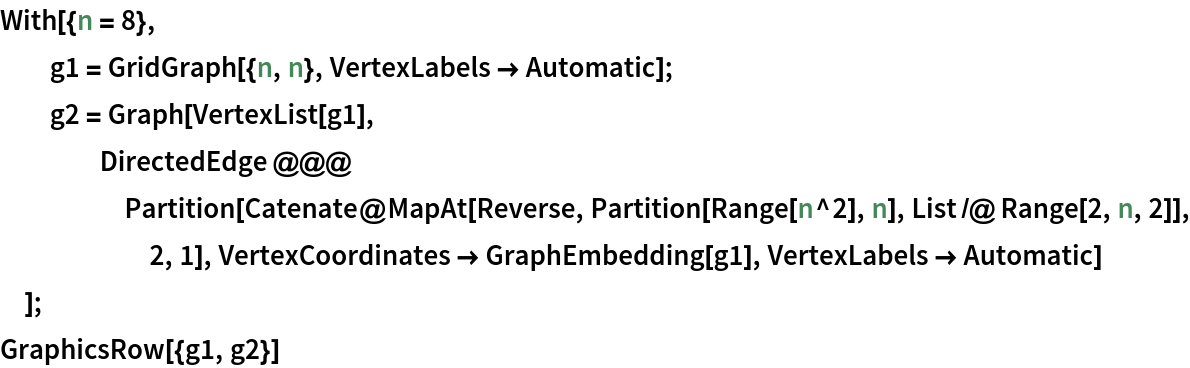Out=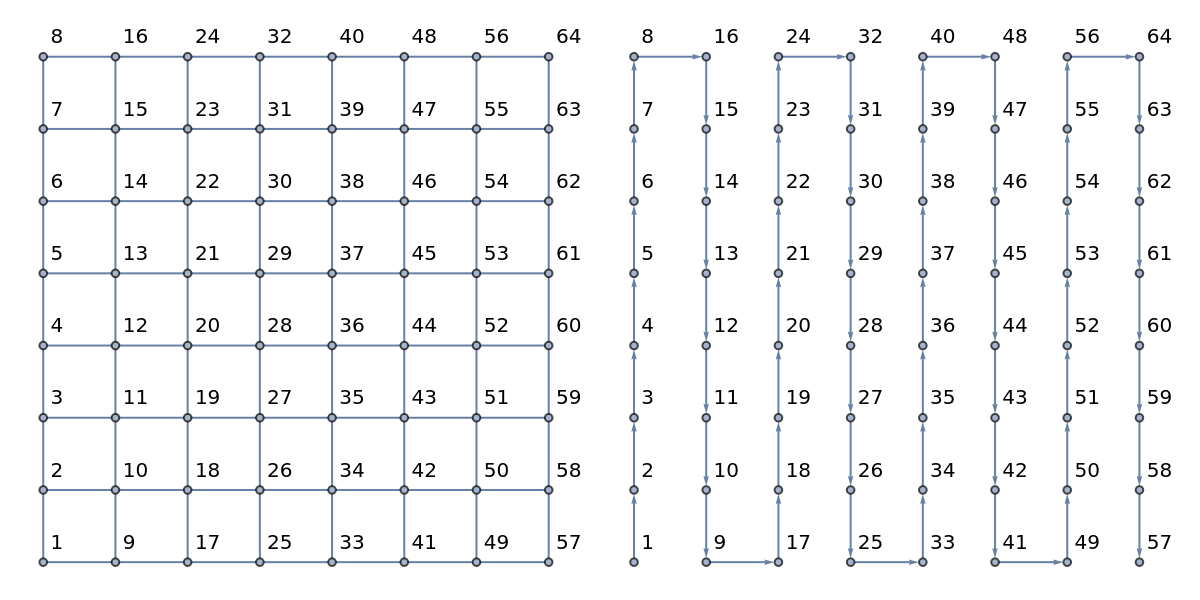Compute an embedding:

 In:=Out=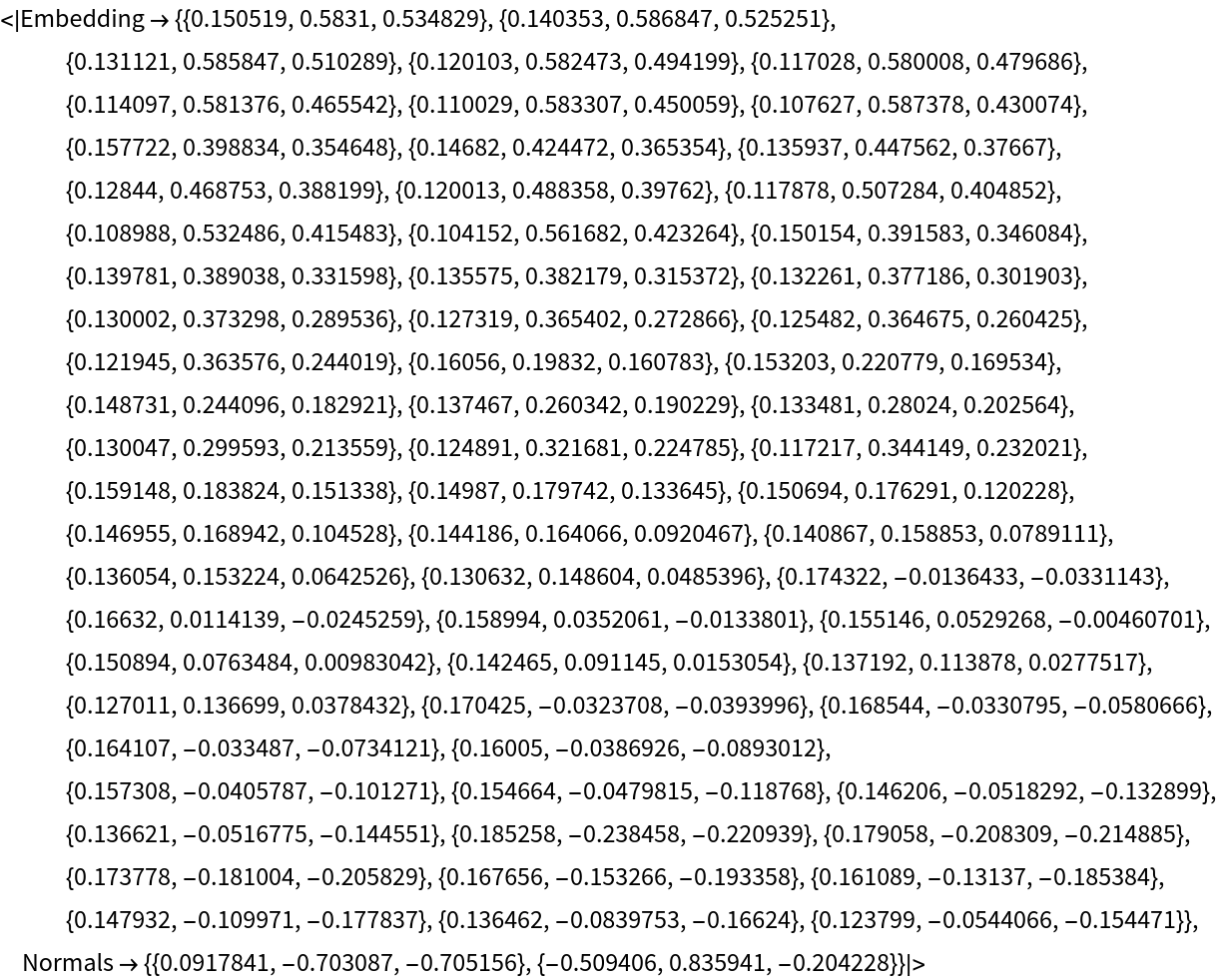View the embedding with the resource function MultiPerspectiveEmbeddingViewer:

 In:=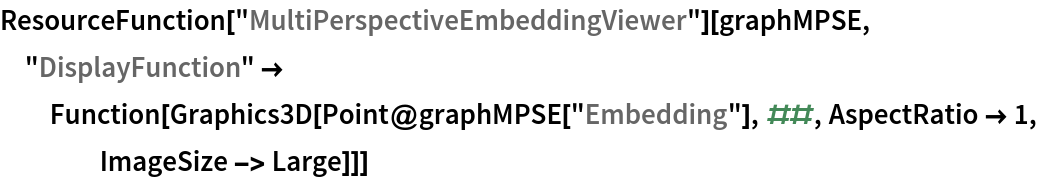Out=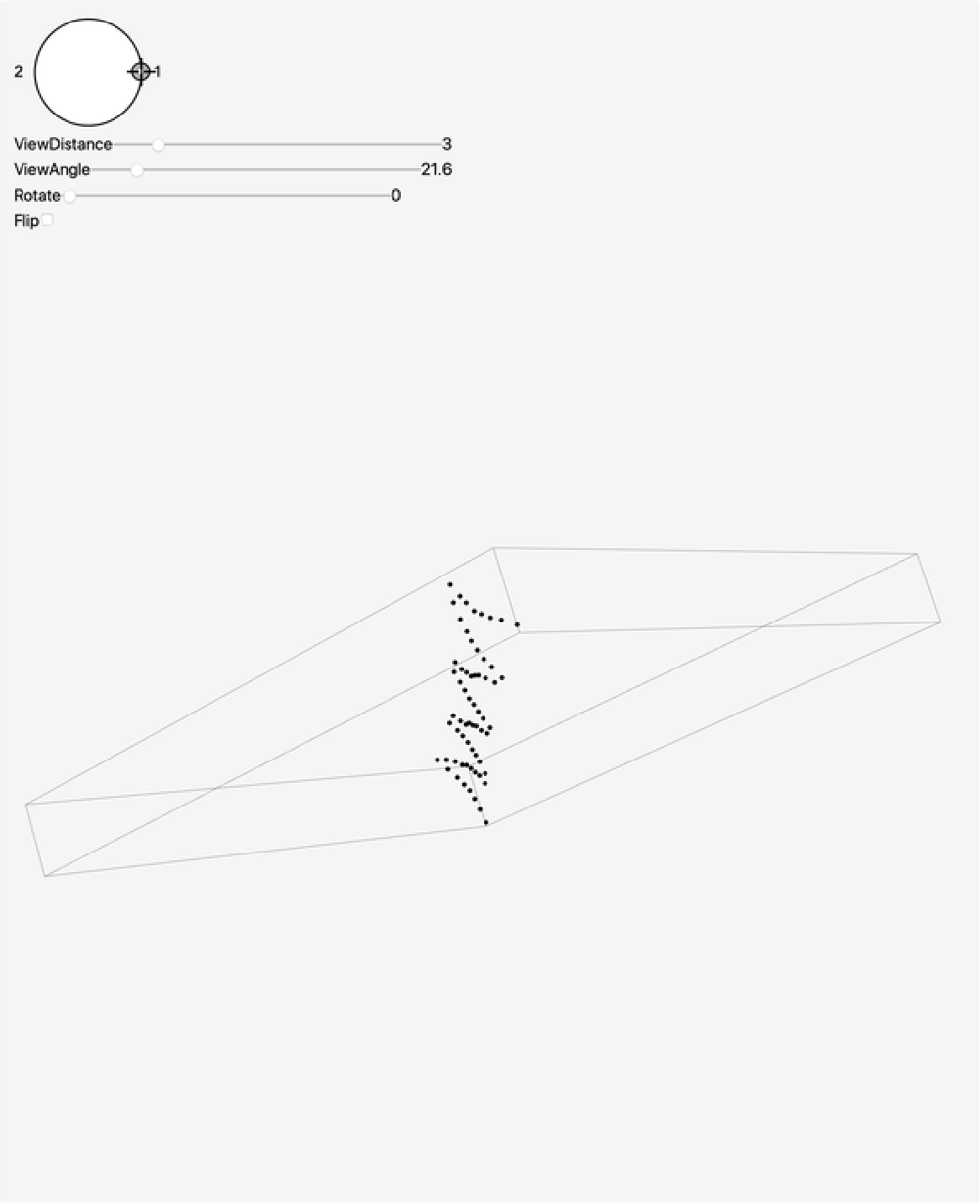Here is a progress of training an embedding for points that look like digits 1, 2 and 3 in 2D from three distance matrices and how they form into their position: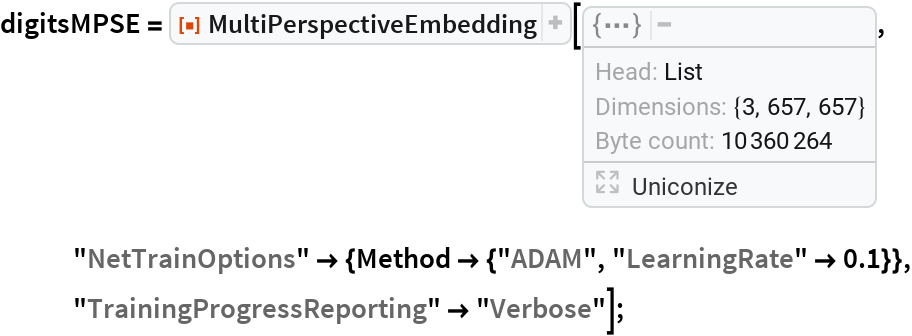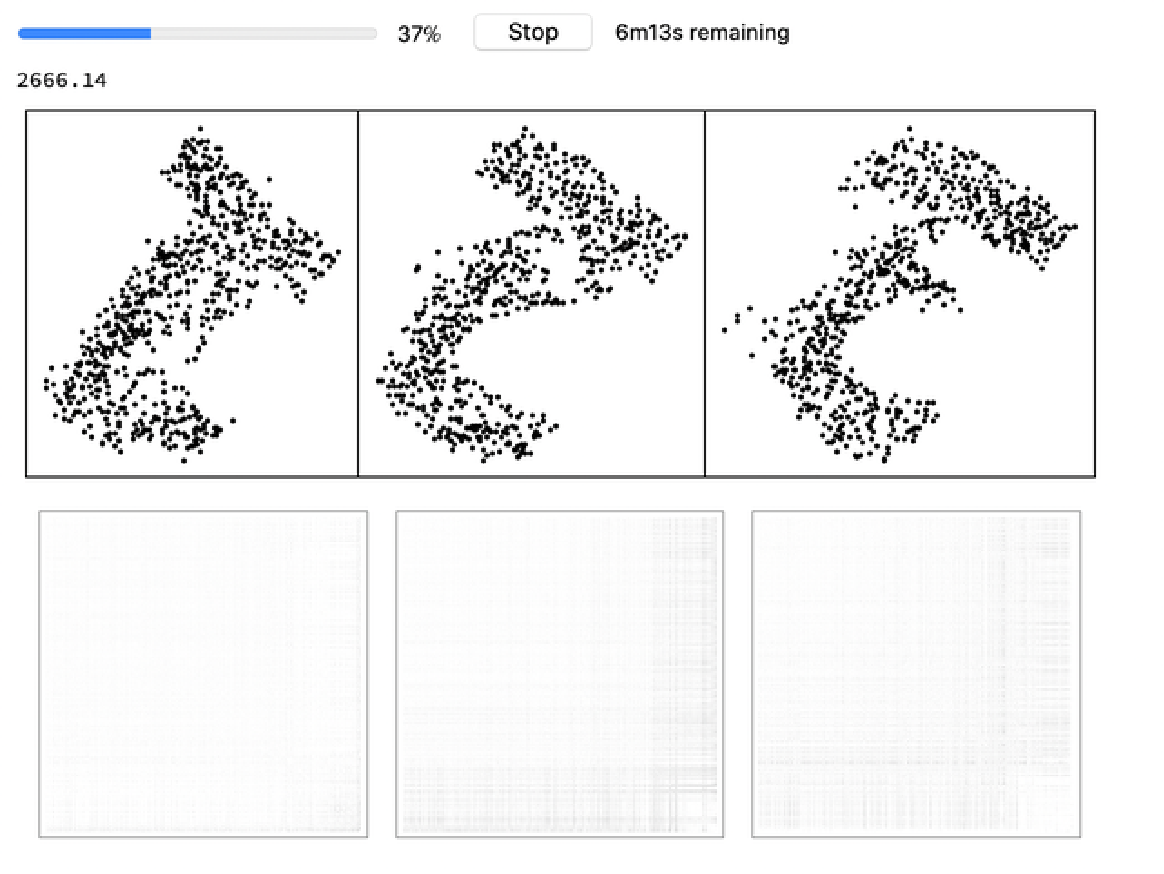View the embedding:

 In:=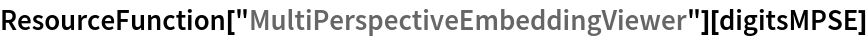Out=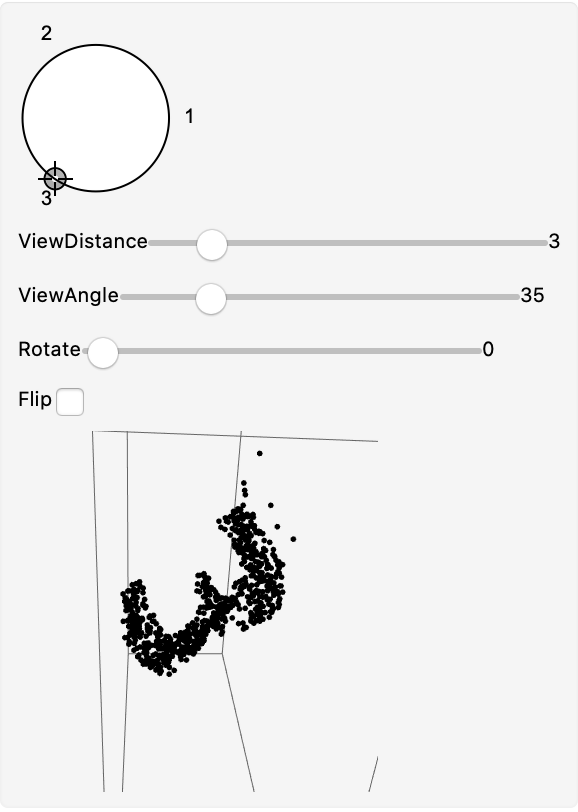### Options

#### NetTrainOptions

Specify custom options for NetTrain, for example MaxTrainingRounds:

 In:=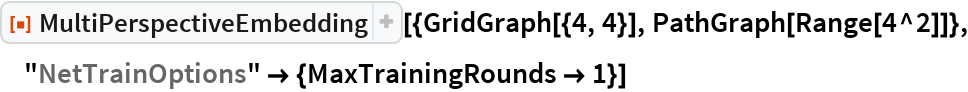Out=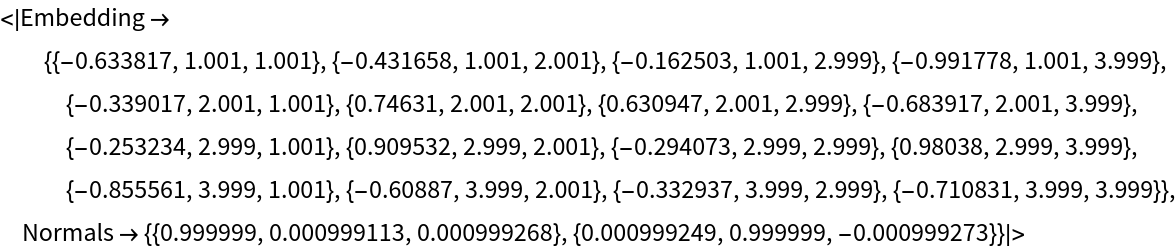#### InitialEmbedding

Specify an initial embedding:

 In:=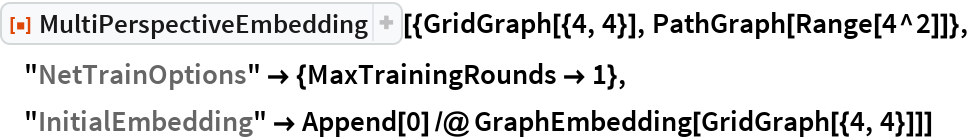Out=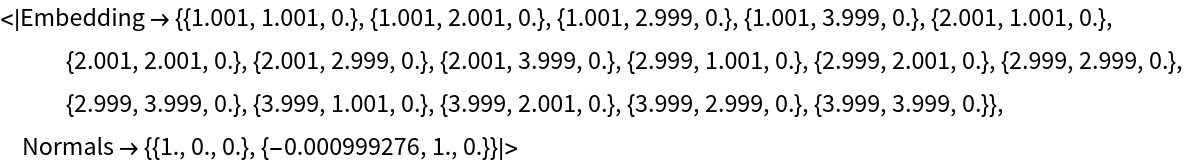#### InitialProjection

Specify initial normal projection vectors using the "InitialProjection" option. The "Normals" key of the resulting association would contain a new projection normal vectors after training:

 In:=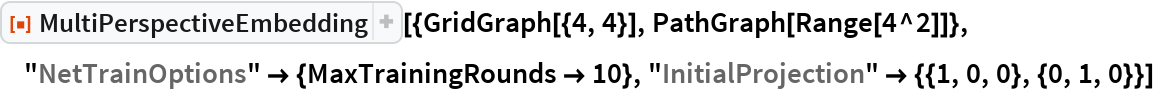Out=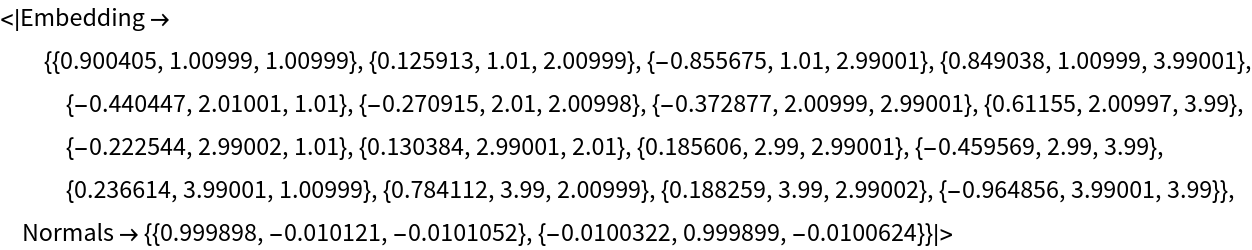#### FixProjection

Disable learning for normal vectors. The "Normals" value stays the same:

 In:=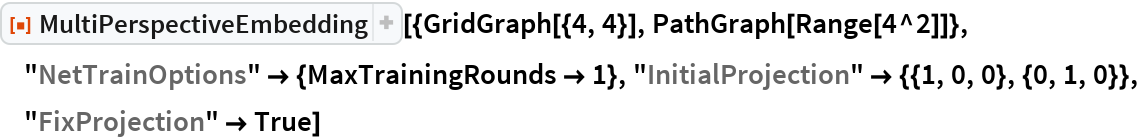Out=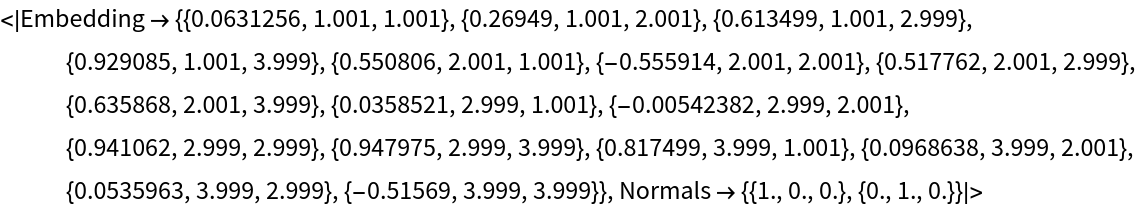#### UseGraphEmbedding

By default, distances are taken to be distances between its GraphEmbeddings: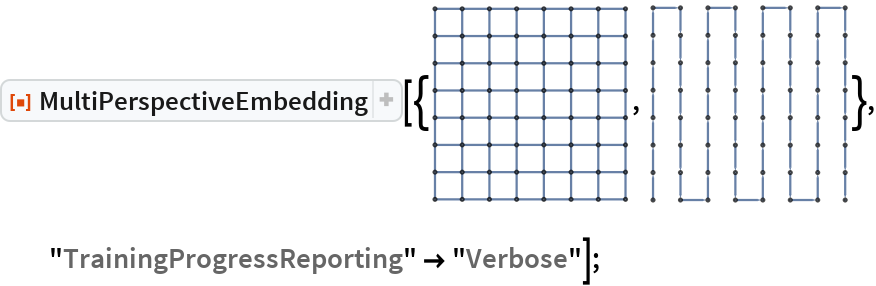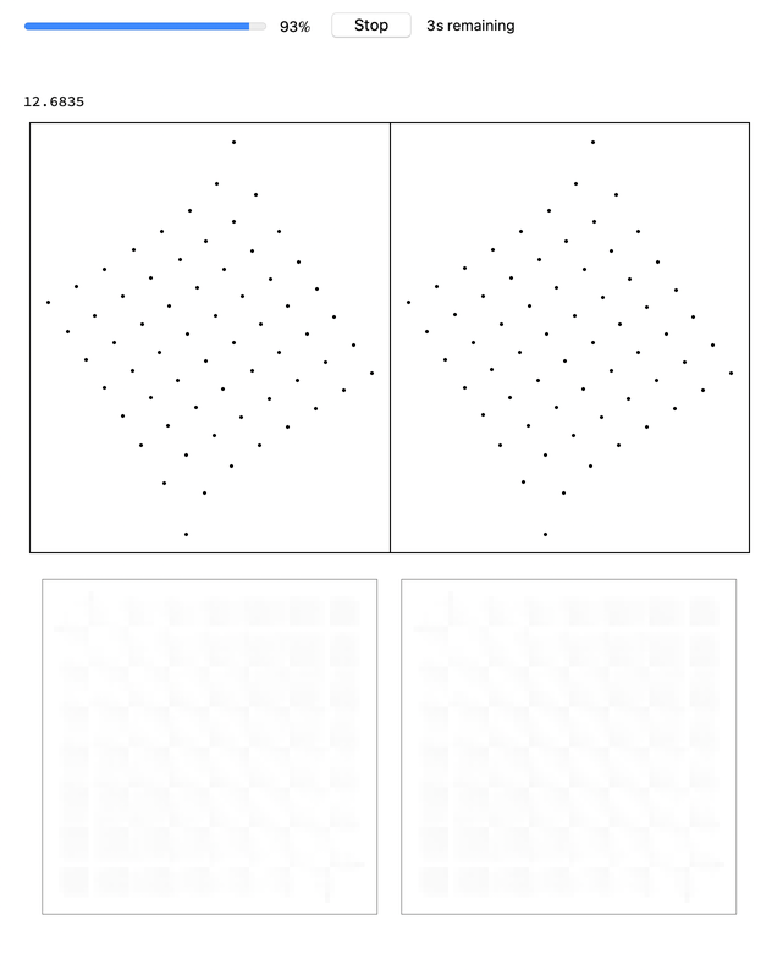UseGraphEmbedding False would use GraphDistanceMatrix instead: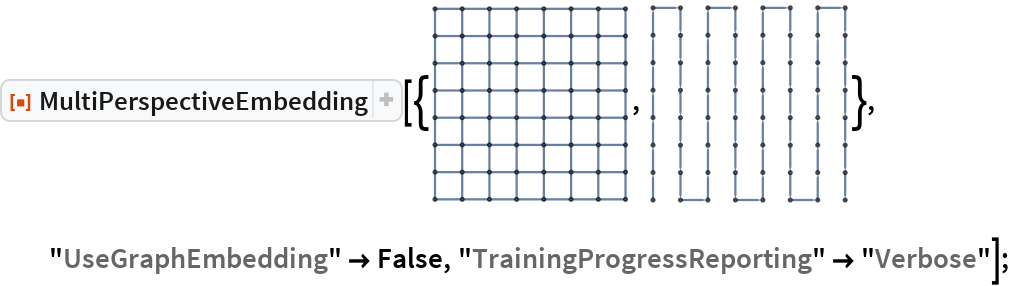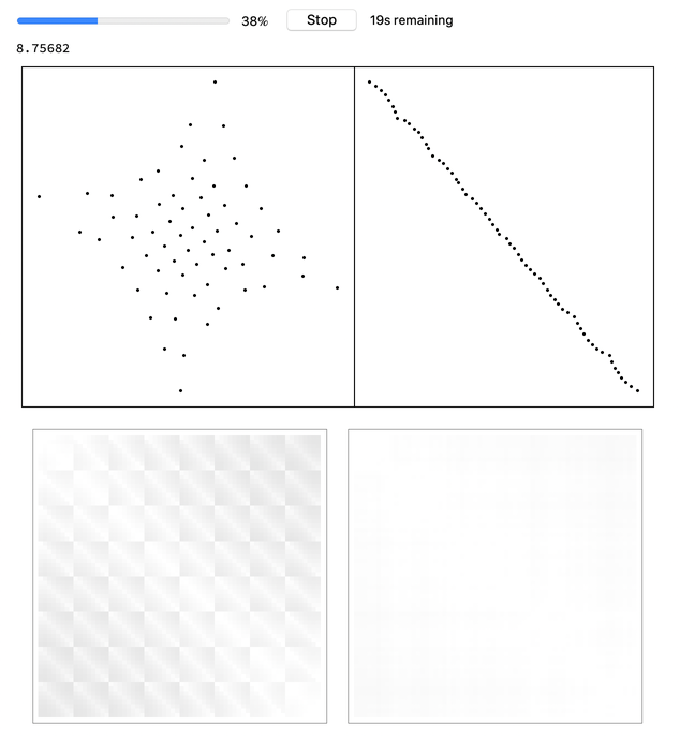#### NormalizeDistances

Without normalizing distances, training may diverge: